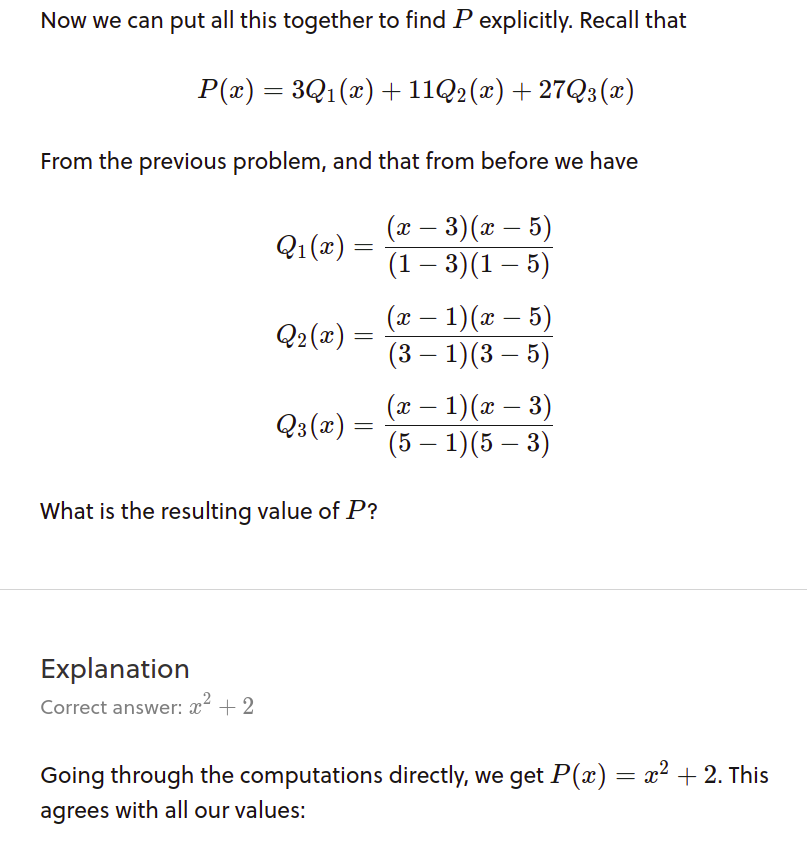# Polynomial Interpolation example

I'm having trouble understanding this result (actually, this whole chapter of the Linear Algebra course really):(a) Where did these fractions come from? The previous sections do not indicate how we got a rational function.

(b) Is the answer given, $x^2 + 2x$ correct? When I multiplied these rational functions by the constants in question I got:

$P(x) = x^2 + 2x - \frac{7}{4}$

EDIT: For part (b) I figured out where I went wrong, just an algebraic error. $x^2 + 2$ is correctly given, I just misread the answer.

I feel like I have no idea what is going on here even though I just finished a class in linear algebra.Note by Jeff Folster
11 months, 2 weeks ago

This discussion board is a place to discuss our Daily Challenges and the math and science related to those challenges. Explanations are more than just a solution — they should explain the steps and thinking strategies that you used to obtain the solution. Comments should further the discussion of math and science.

When posting on Brilliant:

• Use the emojis to react to an explanation, whether you're congratulating a job well done , or just really confused .
• Ask specific questions about the challenge or the steps in somebody's explanation. Well-posed questions can add a lot to the discussion, but posting "I don't understand!" doesn't help anyone.
• Try to contribute something new to the discussion, whether it is an extension, generalization or other idea related to the challenge.

MarkdownAppears as
*italics* or _italics_ italics
**bold** or __bold__ bold
- bulleted- list
• bulleted
• list
1. numbered2. list
1. numbered
2. list
Note: you must add a full line of space before and after lists for them to show up correctly
paragraph 1paragraph 2

paragraph 1

paragraph 2

[example link](https://brilliant.org)example link
> This is a quote
This is a quote
    # I indented these lines
# 4 spaces, and now they show
# up as a code block.

print "hello world"
# I indented these lines
# 4 spaces, and now they show
# up as a code block.

print "hello world"
MathAppears as
Remember to wrap math in $$ ... $$ or $ ... $ to ensure proper formatting.
2 \times 3 $2 \times 3$
2^{34} $2^{34}$
a_{i-1} $a_{i-1}$
\frac{2}{3} $\frac{2}{3}$
\sqrt{2} $\sqrt{2}$
\sum_{i=1}^3 $\sum_{i=1}^3$
\sin \theta $\sin \theta$
\boxed{123} $\boxed{123}$

Sort by:

- 11 months, 2 weeks ago

I believed you're referring to this problem.

The previous problem demonstrates how you can get the coefficients $3,11,27$.

If you substitute the three polynomials $Q_1(x), Q_2 (x) , Q_3 (x)$ as stated into $P(x)$, you get the desired $x^2 +2$.

- 11 months, 2 weeks ago

I know where the coefficients come from, but why are we dividing the polynomials by $(1 - 3)(1 - 5)$, etc.? This process isn't explained at all.

I figured out where my math went wrong, I did get $x^2 + 2$ after all.

- 11 months, 2 weeks ago

Let's say we want to interpolate a set of data points $(a_{1},b_{1}) , (a_{2}, b_{2}) , \dots , (a_{n},b_{n})$ with a polynomial. There are a few real-world applications where this is desirable, so this isn't just an academic exercise. But let's not get into all that now.

If we express the interpolating polynomial $p(x)$ as $p_{0} + p_{1} x + p_{2} x^2 + \dots+ p_{n-1} x^{n-1},$ then we'd have to solve the system of $n$ equations $p(a_{i}) = b_{i}$ for the coefficients of $p(x),$ which is messy and unpleasant.

A better way is to express $p(x)$ as a linear combination of polynomials $Q_{j}(x)$ that are carefully constructed so as to make the interpolation process as smooth as possible.

Imagine it were possible to define a polynomial $Q_{j}(x)$ that satisfies $Q_{j}(a_{i}) = 0$ if $i \neq j$ but $Q_{j}(a_j) = 1.$ In other words, $Q_{j}$ vanishes at all of the $a$'s except for $a_{j}$ where it's 1.

Assuming for the moment that it's possible to do this, then consider the polynomial $p(x) = b_{1} Q_{1}(x) + b_{2} Q_{2}(x) + \dots + b_{n}Q_{n}(x).$ If we plug in $a_{j},$ then we get $p(a_{j}) = b_{1} Q_{1}(a_{j}) + b_{2} Q_{2}(a_{j}) + \dots + b_{n}Q_{n}(a_{j}) = b_{1} \times 0 + \dots + b_{j} \times 1 + \dots + b_{n} \times 0 = b_{j}.$ So the linear combination of these hypothetical $Q$'s with weights given by the $b_{j}$'s, the $y$-coordinates of the data points, is precisely the interpolating polynomial we're after.

We just have to show that the $Q$'s exist. We can do this by constructing them. The polynomial $(x-a_{1}) (x-a_{2}) \dots (x-a_{j-1})(x-a_{j+1}) \dots (x-a_{n})$ is designed to be 0 at every $a$ except for $a_{j}.$ This is almost what we need, but the polynomial isn't 1 at $a_{j}.$

The simple fix is to take the polynomial above and then divide by $(a_{j}-a_{1}) (a_{j} -a_{2}) \dots (a_{j}-a_{j-1})(a_{j}-a_{j+1}) \dots (a_{j}-a_{n}).$ This gives us a formula for $Q_{j}(x)$:

$Q_{j}(x) = \frac{(x-a_{1}) (x-a_{2}) \dots (x-a_{j-1})(x-a_{j+1}) \dots (x-a_{n})}{(a_{j}-a_{1}) (a_{j} -a_{2}) \dots (a_{j}-a_{j-1})(a_{j}-a_{j+1}) \dots (a_{j}-a_{n})}.$

Hopefully, that explains why we divide by $(1- 3)(1-5)$ in the problem you reference above.

In the future, if you have concerns about a problem's wording/clarity/etc., you can report the problem. See how here.

Staff - 11 months, 2 weeks ago